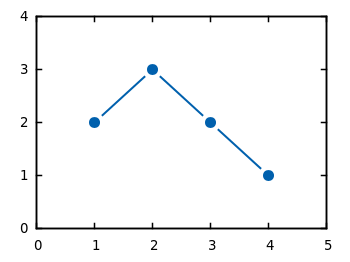# Gnuplotting

Create scientific plots using gnuplot

June 9th, 2010 | 11 Comments

If you have measurement data and like to plot them as points combined by lines, you will probably do that with the `linespoints` plotting style. But for some applications it is required to combine the data points by non-continuous lines to emphasize that the data came from measurements as shown in Fig. 1.Fig. 1 Plot of the data from plotting_data1.dat with non-coninuous lines between its points (code to produce this figure)

In Gnuplot exists no line style that can do this directly. But with a little trick it is very easy to achieve. Since Gnuplot 4.4. there exists the property `pointinterval` (see the documentation) in combination with the plotting style ` linespoints`. This property plots not every single point, but only every second for a value of `2` and so on. But if we use the value `-1` it tells Gnuplot to insert a little gap between the points and the line. The size of the gap can be set by the `pointintervalbox` property.

```set style line 1 lc rgb '#0060ad' lt 1 lw 2 pt 7 pi -1 ps 1.5
set pointintervalbox 3
```

We specify a point interval `pi` of -1 and a point size of 1.5, in addition we set the the gap to a point size of 3. Now we can plot our data with the `linespoints` style.

```plot 'plotting_data1.dat' with linespoints ls 1
```

Using the same data as in the first plot of the gnuplot basics tutorial Plotting data we will get Fig. 1 as a result.